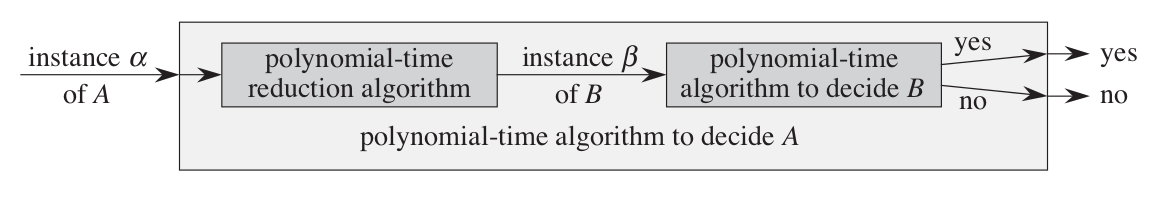CLRS-ch34 NP-Completeness

Intro

class P

• problems are solvable in polynomial time

class NP

• problems are verifiable in polynomial time

class NPC

• problems in NP and is as hard as any problem in NP
• 3 concept showing a problem is NPC
1. decision problems
2. reductions
3. the first NP-complete problem

Decision problems vs. optimization problems

• optimization problems : find a feasible solution with the best value.
• decision problems : answer is simply “yes” or “no”
• Relationship : decision is no harder

Reductions

• $A \longrightarrow B$
• If B polynomially solvable, than A solvable
• If A polynomially unsolvable, than B unsolvable as well34.1 Polynomial time

Abstract Problems

• a binary relation (function) on a set $I$ of instances and a set $S$ of solutions

Concrete Problems

• problems whose instance set $I$ is the set of binary strings

Polynomial time solvable

• exist an algorithm to solve a concrete problem in $O(n^k)$

Encodings

• a mapping e from the set of abstract object to the set of binary strings (abstract pro instances –> concrete pro ins)
• extend the definition of class P into abstract problems
• Abstract Pro $\longrightarrow$ Encode $\longrightarrow$ Concrete Pro $\longrightarrow$ Algorithm Solve
• depending on the encoding, the algorithm may runs in either polynomial or superpolynomial time.

• polynomially related encodings

• exist a polynomial-time algorithm can compute the en-coding $e_2 (i)$ from the encoding $e_1 (i)$, and vice versa.
• Only when two encodings $e_1$ and $e_2$ of an abstract problem are polynomially related, whether the problem is polynomial-time solvable or not can be independent of which encoding we use.

Therefore

Assume reasonable/standard encoding $< G >$

• encoding of an integer is polynomially related to its binary representation
• encoding of a finite set is polynomially related to its encoding as a list of its elements
• derive encodings of other object

so that the choice of encoding has no effect on whether the abstract problem is polynomial-time solvable.

A formal language framework

• alphabet $\Sigma$ a finite set of symbols $\{ 0,1 \}$
• language $L$ over $Σ$ is any set of strings made up of symbols from $Σ \ \{ 10, \ 11, \ 101 … \}$
• $\Sigma ^*$ the language of all strings over $Σ$

view instances for any decision problems Q as a language L over $Σ = \{ 0 ,1 \}$

Accepted

• The language L accepted by an algorithm A $L = \{ x \in \{0,1\}^* : A(x) = 1 \}$
• Reject : A(x) = 0
• may runs forever if doesn’t accept

Decided

• The language L decided by an algorithm A
• if every binary string in L is accepted by A (output 1) and every binary string not in L is rejected ( output 0)
• $x \in L$ –> A(x) = 1, $x\notin L$ –> A(x) = 0

Complexity class P

$P = \{ L \subseteq \{ 0,1 \}^{*}$ : exists an algorithm A that decides L in polynomial time $\}$

34.2 Polynomial-time verification

algorithms verify membership from a certificate in languages

Verified

• The language L verified by an algorithm A $L = \{ x \in \{ 0, 1 \}^ :$ there exist $y \in \{ 0,1 \}^$ such that A( x,y ) = 1 $\}$

Complexity class NP

$NP = \{ L \subseteq \{ 0, 1 \}^ : L = \{ x \in \{ 0,1\}^$ : there exists a certificate y with |y| = $O(|x|^c)$ such that A(x,y) = 1 $\} \}$

$P \subseteq NP$

Complexity class co-NP

• set of languages L such that $\bar L \in NP$
• Since P is closed under complement, $P \subset NP \cap co-NP$

Four possibilities for relationship among complexity class34.3 NP-completeness and reducibility

Reducibility

• if $Q \longrightarrow \ reduce \ \longrightarrow Q’$ , $Q$ is “no harder to solve” than $Q’$
• $Q \le_p Q’$ ( no more than a polynomial factor harder )

NP-completeness

1. $L \in NP$
2. $L’ \le_p L$ for every $L’ \in NP$. (hardest)

Theorem 34.4

• If any NP-complete problem is polynomial time solvable, then P = NP
• If any problem in NP is not polynomial-time solvable, then all NP-complete problems are not polynomial-time solvable.Circuit satisfiability

Given a boolean combinational circuit composed of AND, OR, and NOT gates, is it satisfiable?

34.5 belongs to NP

34.6 NP-hard

• basic idea : represent the computation of A(algorithm verify L) as a sequence of configurations.
• Each configuration is mapped to the next configuration by a boolean combinational circuit M. The output is a distinguished bit in the working storage.
• The reduction algorithm F constructs a single combinational circuit that computes all configurations produced by a given initial configuration.
•34.5 NP-complete problems